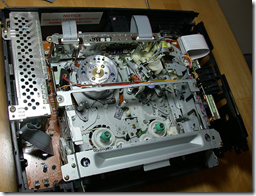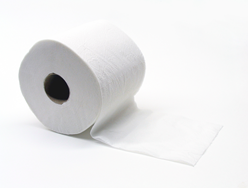# The Mathematics of Toilet Rolls

Posted by Graham Wheeler on Sunday, December 27, 2009

In the late 1980s I was contracted to write some software for a company that produced video-based educational systems. They had video cassette machines that had been modified to interface with a PC, which could send instructions to the VCRs such as play, stop, fast forward, and rewind. The educational programs consisted of short recorded segments which typically ended with a question, and based on the answer the user provided they wanted to continue play at different portions of the tape. The software they wanted me to write was meant to be able to be given a current position (i.e. play time) and advance to a new position. For example I might be told that they are at minute 8 currently and want to resume play at minute 12.Without going into all the detail, I can tell you this was a Very Hard Problem. There was no way to tell where you were on the tape without actually playing, and if you are familiar with VCRs (which are rapidly going the way of the dinosaur) you may be aware that engaging the play head takes a few seconds. There were variations in motor speed, tape stretching, and all sorts of other variables to take into account. The approach I used was to try to calculate the “optimal” fast forward time, shorten that a bit, and then narrow down to the exact position with a modified binary search (or, to put it more crudely, “trial and error”).

We got the system to work although it was a pretty crude affair but before anything shipped new VCRs came out that recorded “time code” on the tape and had much more precise electronics essentially supporting just this functionality we had duct-taped together, and so it was all for naught (and not long after CD-ROMs came and made it all redundant anyway).

I don’t remember all the math that I worked out for this system, but it is easy enough to recall the basic parameters. There was the diameter of the empty plastic spools, the diameter of a full spool, and the playing time of the tape (typically 120 minutes), which was a linear function of the length (assuming an unstretched tape :-))

While playback happened at a constant speed, as the tape ran through an independent roller mechanism unaffected by the spool state, fast-forwarding and rewinding happened at a variable rate, as it was controlled by the motors driving the spool, and the spool diameters changed.All in all, a nasty business, and we are better off without tape! But there is still a common household item with some similar characteristics, namely the toilet roll. So, here are some problems surrounding toilet rolls to think about. To save you having to measure your toilet rolls here are some typical measurements from mine (Charmin 2 ply from Costco) - spool diameter 45mm, roll diameter 120mm, 250 x 101mm sheets = 25250mm length.

• how thick is each sheet of paper? (and thus, how many times does it go around the spool?)
• what would the diameter of a roll be that went all the way around the earth (assuming the earth’s diameter is 12,756.32km)?
• what if it went around the earth but 1m higher than in the example before? How long would the roll be? (you may recognize this as a familiar puzzle involving rope but I thought I’d sneak it in here!)

(BTW no less a figure than Donald Knuth has considered toilet paper worthy of investigation, as evidenced here).

If we call the paper thickness $$t$$, the radius of the spool $$r_s$$ and the radius of the roll (spool plus paper) $$r_r$$, then it should be obvious that the number of winds $$n$$ is:

$$n = \frac{r_r - r_s}{t}$$

If we approximate the roll as a number of concentric circles rather than a spiral, then the innermost roll has length $$2\pi r_s$$, and the next roll has length $$2\pi (r_s+t)$$, and so on; each successive roll is $$2\pi t$$ longer than the prior one, and the final roll has length $$2\pi r_r$$.

Because each successive roll is longer by a constant amount, we have an arithmetic sequence. Recall that the sum of an arithmetic sequence is given by $$\frac{n}{2}(a_1 + a_n)$$, where $$n$$ is the number of terms, $$a_1$$ is the first term, and $$a_n$$ is the last term. So the length of the entire roll is given by:

$$l = \frac{n}{2} ( 2\pi r_s + 2 \pi r_r) = \pi \frac{r_r^2 - r_s^2}{t}$$

Thus my toilet paper is about 0.38 mm thick - quite luxurious!

For the second problem it is reasonable to assume $$r_s = 0$$, to give us the simpler formula:

$$l \approx \pi \frac{r_r^2}{t}$$

or:

$$r_r \approx \sqrt{\frac{l t}{\pi}}$$

or:

$$r_r \approx \sqrt{d t}$$

where $$d$$ is the earth’s diameter. So for my paper this would be about 70 meters. If I had a cheaper brand of one ply paper this might be considerably less.

I’ll leave the last question as an exercise but it should be fairly clear that the difference in $$l$$ in this case is quite small and so the extra radius needed for the toilet roll is very small.